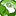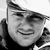QlikView App Dev

Discussion Board for collaboration related to QlikView App Development.

Announcements
QlikWorld 2022, LIVE in Denver CO., May 16-19, 2022. REGISTER NOW TO RECEIVE EARLY BIRD PRICING
cancel
Showing results for
Did you mean:Contributor

Set Analysis expression using multiple if statements ?

How can write this if else formula using set analysis ?

If label = 'A' and client = 'X' then  Sum(measurefield1)/If label = 'B and client = 'Y' then  Sum(measurefield2)

If label = 'C' and client = 'X' then  Sum(measurefield3)/If label = 'D' and client = 'X' then  Sum(measurefield4)

If label = 'E' and client = 'X' then  Sum(measurefield5)

If label = 'F' and client = 'X' then  Sum(measurefield6)

Labels (2)

• Set Analysis

4 RepliesMVP
I think a PickMatch would be a good choice in this scenario.

Sum(Pick (Match(Label&Client,'AX','BY' ,'CX', 'DX' , 'EX') ,measurefield1, measurefield2, measurefield3, measurefield4, measurefield 5))

Plees ekskuse my Swenglish and or Norweglish spelling misstakesContributor
Author
How can I calculate the division(Sum(Measure1)/Sum(Measure2) using multiple if conditions as I mentioned in my initial post ?Specialist II
Since the alphabets match the field number in your requirement create a variable like the below

Variable1 = 'MEASURE'&( (ord('\$(=FIELD)') +1)-65)

Expression : Sum( \$(variable1 ))

The measure field will change based on the selection you makeContributor
Author
How can I calculate the division using multiple if statements ?Community Browser